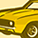cancel
Showing results for
Did you mean:
cancel
Showing results for
Did you mean:
Developer forum
GDL coding questions, Python and add-on development using the API Dev Kit.

## Length/Size of one of the sub-arrays of a multi-dim arrayEnthusiast
Hi
Is there a way to get the length/size of a sub-array of a multi-dimensional array?
```DIM myArray[][]
myArray = 500
myArray = 510
myArray = 600
myArray = 610
myArray = 620
myArray = 700
myArray = 710
myArray = 720
myArray = 730```
VARDIM1(myArray) !gives 4, but I need the size of just myArray, which is 2

I tried VARDIM2(myArray) also but it doesn't give the required output.
32 REPLIES 32Ace
We started here (I started) the thread called Open Source GDL Project - where users can exchange GDL scripts, ideas, macros and develop new tools in open source manner.Enthusiast
Ah, okay.Graphisoft
I think this might help:
```dict d
dim EMPTYARRAY[]

d.array.subarray = EMPTYARRAY
d.array.subarray = EMPTYARRAY

d.array.subarray = 11
d.array.subarray = 21
d.array.subarray = 22

print d, vardim1(d.array.subarray), vardim1(d.array.subarray)
```
dushyant wrote:
In that case, multi-dim arrays might not be helpful for what I am trying to do. Thanks guys for the help.

Can dictionaries be looped through?
Péter Baksa
Software Engineer, Library as a Platform
Graphisoft SE, BudapestLatest solutions

Most liked posts